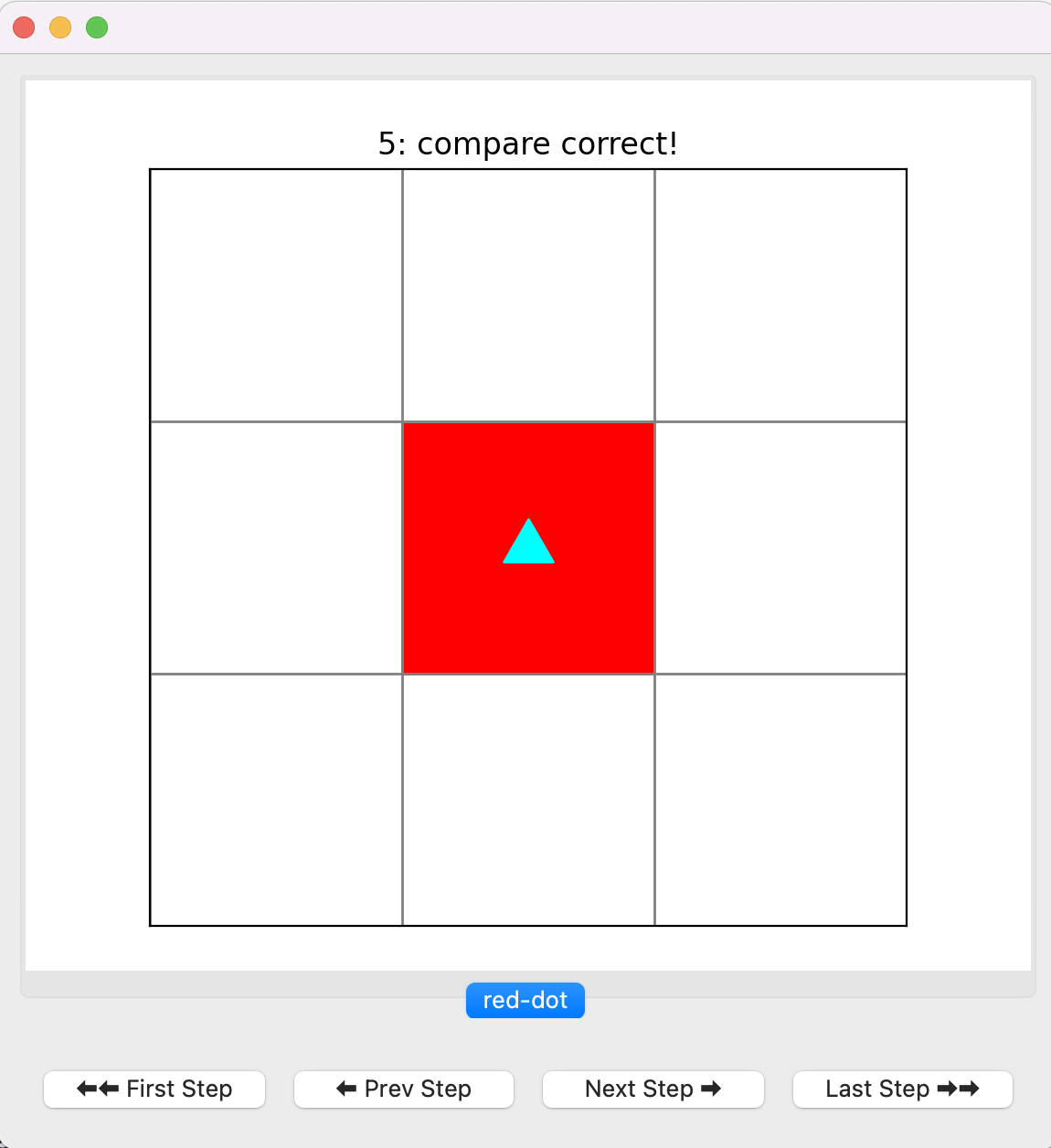Computer Science

# Bit reference

The `byubit` library helps you learn to program by drawing pictures and solving puzzles. Bit works in a “world” of squares:You can think of Bit as a robot, in the shape of a triangle. Bit has a position and a direction. In the picture above, Bit is at the bottom left, facing right.

## Installing byubit

See the guide on installing Bit.

## Importing Bit

To use Bit, you always need to import it using the following:

``from byubit import Bit``

## Running Bit in an empty world

You can run bit in an empty world using:

``````@Bit.empty_world(5, 3)
def main(bit):
# write some code here

if __name__ == '__main__':
main(Bit.new_bit)``````

This runs Bit in a 5 by 3 world.

## Running Bit in a world

You run bit in a world using:

``````@Bit.world(world-name)
def main(bit):
# write some code here

if __name__ == '__main__':
main(Bit.new_bit)``````

For example, you can run Bit on the `red-dot` world using a function called `red_dot` like this:

``````@Bit.world'red-dot')
def red_dot(bit):
# Implement me!
pass

if __name__ == '__main__':
red_dot(Bit.new_bit)``````

This loads a world from a file called `red-dot.start.txt`. You can then write code in the function to control Bit.

## Moving Bit

You can move and turn Bit using the following functions:

• `bit.move()` — move forward one space
• `bit.right()` — turn right (without moving)
• `bit.left()` — turn left (without moving)

This example moves Bit a few spaces and turns:

``````bit.move()
bit.left()
bit.move()
bit.right()
bit.move()``````

## Painting colors

You can use the following functions to change the colors of squares:

• `bit.paint(color)` — paint the color of the current square; valid colors are ‘red’, ‘green’, and ‘blue’
• `bit.get_color()` — returns the color of the current square
• `bit.erase()` — erases the color of the current square

This example paints the current square red:

``bit.paint('red')``

This example gets the color of the current square and stores it in a variable:

``current_color = bit.get_color()``

## Checking colors

You can check the color of the current square:

• `bit.is_red()` — returns true if the current square is red
• `bit.is_blue()` — returns true if the current square is blue
• `bit.is_green()` — returns true if the current square is green
• `bit.is_empty()` — returns true if the current square is white

This example moves until the current square is not red:

``````while bit.is_red():
bit.move()``````

## Checking if a square is clear

Bit can’t move if the square in front of it is black, or if it reaches the end of its world. You can use the following methods to check squares near Bit:

• `bit.front_clear()` — checks if the square in front of Bit is clear (not black, not the end of the world)
• `bit.right_clear()` — checks if the square to the right of Bit is clear
• `bit.left_clear()` — checks if the square to the left of Bit is clear

This example moves Bit until it reaches the end of the world:

``````while bit.front_clear():
bit.move()``````

## Stepping

You can use the graphical Bit interface to step through every command that you give to Bit. By default, the interface runs your entire program and stops at the end:This shows that you are running the `red-dot` world and that your finish state matches the solution (“compare correct!”). You can use the `Prev Step` and `Next Step` buttons to go backwards and forward. You can use the `First Step` and `Last Step` buttons to go to the beginning or end.

## Snapshots

You can create a snapshot of the current bit state using:

• `bit.snapshot(name)` — creates a snapshot with the given name

Imagine you are trying to move Bit to the top right corner. This example creates a snapshot called `halfway` that stops after Bit should have reached the right side.

``````while bit.front_clear():
bit.move()
bit.snapshot('halfway')
bit.left()
while bit.front_clear():
bit.move()``````

This is helpful for debugging because you can go right to a particular point of your code instead of stepping through everything one command at a time.

## Summary

``````@Bit.run(world-name)
def function_name(bit):
# write some code here``````
• Moving
• `bit.move()` — move forward one space
• `bit.right()` — turn right (without moving)
• `bit.left()` — turn left (without moving)
• Painting
• `bit.paint(color)` — paint the color of the current square; valid colors are ‘red’, ‘green’, and ‘blue’
• `bit.get_color()` — returns the color of the current square
• `bit.erase()` — erases the color of the current square
• Checking Colors
• `bit.is_red()` — returns true if the current square is red
• `bit.is_blue()` — returns true if the current square is blue
• `bit.is_green()` — returns true if the current square is green
• `bit.is_empty()` — returns true if the current square is empty
• Checking if a Square is Clear
• `bit.front_clear()` — checks if the square in front of Bit is clear (not black, not the end of the world)
• `bit.right_clear()` — checks if the square to the right of Bit is clear
• `bit.left_clear()` — checks if the square to the left of Bit is clear
• Snapshots
• `bit.snapshot(name)` — creates a snapshot with the given name